﻿ The Dynamic International Optimal Hedge Ratio

### The Dynamic International Optimal Hedge Ratio

Xiaochun Liu, Brian JacobsenOPEN ACCESSPEER-REVIEWED

## The Dynamic International Optimal Hedge Ratio

Xiaochun Liu1,, Brian Jacobsen2

1Department of Economics, Emory University, Atlanta, United States

2Chief Portfolio Strategist, Wells Fargo Funds Management, LLC, Milwaukee, United States

### Abstract

Instead of modeling asset price and currency risks separately, this paper derives the international hedge portfolio, hedging asset price and currency risk simultaneously for estimating the dynamic international optimal hedge ratio. The model estimation is specified in a multivariate GARCH setting with vector error correction terms and estimated for the commodity and stock markets of the U.S., the U.K., and Japan.

### At a glance: Figures

1
Prev Next

• Liu, Xiaochun, and Brian Jacobsen. "The Dynamic International Optimal Hedge Ratio." International Journal of Econometrics and Financial Management 2.3 (2014): 82-94.
• Liu, X. , & Jacobsen, B. (2014). The Dynamic International Optimal Hedge Ratio. International Journal of Econometrics and Financial Management, 2(3), 82-94.
• Liu, Xiaochun, and Brian Jacobsen. "The Dynamic International Optimal Hedge Ratio." International Journal of Econometrics and Financial Management 2, no. 3 (2014): 82-94.

 Import into BibTeX Import into EndNote Import into RefMan Import into RefWorks

### 1. Introduction

Globalization has brought additional choices of investing instruments, but that additional choice can also bring confusion. For a U.S.-based company that has a spot-asset risk exposure, management may be interested in not only hedging dollar-denominated risk, but also foreign exchange risk. For example, a U.S. firm that has a copper asset may want to contract with a U.K.-based firm to sell the asset. This not only exposes the parties to the price risk of copper, but also currency risk. For the U.S. firm, a futures contract on copper can be sold in either the U.S. or U.K. This may hedge the price risk of copper, but there is still the outstanding issue of the foreign exchange risk. At any given time, these alternative hedging “bundles” (commodity and currency futures) should be priced according to the law of one price. Transaction costs can drive a wedge between the theoretical prices that should prevail. In this paper, we examine which hedging bundle is optimal. In a very real way, this is equivalent to the covered versus uncovered interest rate parity theory of exchange rates, but instead of interest rates, we use the returns on commodities.

Despite the increased importance of international investing, the optimal hedge ratio is rarely estimated between countries’ markets. A number of studies estimate the domestic optimal hedge ratio in commodity markets, currency markets, and financial markets.{1} There are a few notable studies regarding the optimal hedge ratio estimation between international financial markets.{2} Financial market liberalization has stimulated demand for international hedging. In addition to the enormous growth in the hedge fund industry, importers, exporters and multinational corporations utilize financial derivative markets to hedge risks across countries and/or between international markets. Growing demand for international hedging has led to assets being traded in different countries’ markets with more than one currency.

Currency risk plays a significant role in international hedging. De Santis and Gérard (1998) find that currency risk is priced on several major developed stock markets. Antell and Vaihekoski (2007) argue that currency risk can play a very important role in many small developed and/or emerging markets. Similar results have been found for small developed markets (see, Vaihekoski, 2007) and for emerging markets (see, Jacobsen and Liu, 2008; Phylaktis and Ravazzolo, 2004). Consequently, the volatility of currency should be considered in international hedging.

Previous studies, (e.g., Kerkvliet and Moffett (1991), among others), investigate the hedging portfolio where an agent hedges the currency risk of a foreign denominated asset in the currency derivative markets separate from the risk of the underlying asset. Gardner and Stone (1995) have pointed out a deficiency of conducting two hedging operations, namely that the optimal solutions can be highly unstable due to the poor forecasting power of currency derivatives, such that the international hedging portfolio might bear double risks in both foreign asset markets and currency derivative markets. On the one hand, the investor must determine two sets of hedge ratios: one set for the optimal weights of the original foreign assets and the other for the hedging of currency risk; on the other hand, the investor must pay two sets of transaction fees.

This study proposes an alternative approach to deriving the international portfolio hedge without involving an additional hedge operation in the currency derivative market. To accomplish this, we simultaneously hedge the currency risk within the same transaction being used to hedge the spot market risk via the foreign futures contracts. In other words, both asset and currency risks are hedged in one operation.

This paper is structured as below. Section II reviews related literature. Section III develops the simultaneous hedge theory. Section IV specifies empirical econometric models. Section V describes data. Section VI reports empirical results. Section VII concludes this paper.

### 2. Related Literature

This paper extends the mean-variance expected utility framework to derive the international optimal hedge ratio.{3} There are, numerous methods for determining the optimal hedge ratio: minimum variance, maximum Sharpe ratio, and minimum generalized semi-variance.{4} Interestingly, Lee et al. (2006) and Kroner and Sultan (1993) document that under martingale and joint-normality assumptions, various optimal hedge ratios are identical to the minimum variance hedge ratio. However, within the context of simultaneous hedging, the IOHR formulation of this study is not equivalent to those approaches.

We use a multivariate Generalized Autoregressive Conditional Heteroscedasticity model with vector error correction terms (VEC-MGARCH) to estimate the simultaneous IOHR. Many previous studies capture the time-varying feature of the second moments of financial time–series using GARCH models.{5} These studies report that the optimal hedge ratio is time-varying if the true joint distribution of spot and futures returns is changing over time.

### 3. Hedge Theory

Let st and ft* be changes in the spot market prices denoted in domestic currency and in the futures prices denoted in foreign currency at time t, respectively. Also, let et be the exchange rate at time t in domestic currency per unit of foreign currency. Suppose an agent invests in an international hedge portfolio consisting of being long one unit of the spot asset and short h units of the corresponding foreign futures assets priced in the foreign currency. The random cash flow to this hedged portfolio, Π, is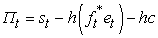(1)

where c is the fixed transaction cost per futures contract. Assume the agent has constant absolute risk aversion utility defined over cash flows of the hedged portfolio. The agent’s problem is to choose an optimal h to maximize the expected utility. If the portfolio return is normally distributed conditional on currently available information, Ωt-1, this problem is equivalent to maximizing the following mean-variance expected utility function: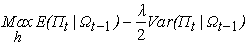(2)

where, λ is the coefficient of absolute risk aversion,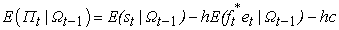, and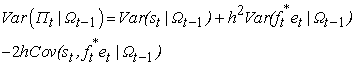.

Solving this optimization problem for equation (2), we obtain the international optimal hedge ratio as h*. The notation of conditional information, Ωt-1 is suppressed in the rest of this paper for simplicity.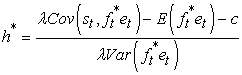(3)

The exact variance and covariance formulation of product of random variables in Equation (3) was originally developed in Bohenstedt and Goldberger (1969) as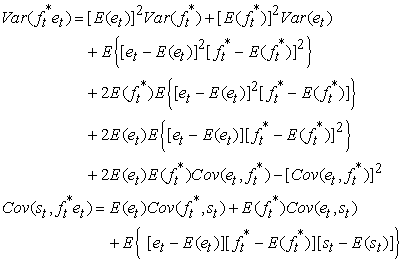Its application in a different setting can be found in Kerkvliet and Moffett (1991), who studied the hedging of uncertain currency cash flow.

Equation (3) can be compared to a purely domestic hedge: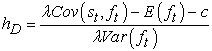(4)

Equations (3) and (4) differ in terms of the futures contract: does any covariance between the exchange rate and the futures contract price help determine the optimal hedge ratio? We empirically test this relation in the section 5.

McCurdy and Morgan (1988) and Kroner and Sultan (1993) show the empirical evidence that the futures rate follows a martingale. The martingale property implies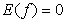, but we will not assume that property for purposes of empirical testing.

Higher transaction costs reduce the international optimal hedge ratio, as expected. However, Jickling (2006), in the report of Congressional Research Service for Congress, states a relatively small transaction cost for derivative markets, which is between 0.01% and 0.003% of the transaction value. Moon, et. al. (2009) reports an even lower transaction cost around 0.00072% in the KOSTAR futures market. To be conservative, we will assume a transaction cost of 0.01%.

This study makes an assumption of λ=4, based on the main findings in the literature, e.g. Grossman and Shiller (1981), Kroner and Sultan (1993), Chou (1988), and Poterba and Summers (1986).

If the futures asset price is uncorrelated with the exchange rate, or the exchange rate is fixed, the international optimal hedge ratio is identical to the traditional domestic optimal hedge ratio. In other words, if the law of one price applies continuously, such that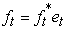, then the domestic and international hedge ratios would be identical. Furthermore, a negative correlation between the exchange rate and the foreign futures asset price leads to a larger hedge ratio than if there is a positive correlation, everything else being held constant. The correlation between the spot price and the exchange rate, nonetheless, has no direct effect on the hedge ratio.

### 4. Model Specification

In this study, a multivariate GARCH model with vector error correction terms (VEC-MGARCH) is specified to estimate the optimal hedge ratio over three hedging horizons. The optimal hedge ratio is adjusted continuously based on conditional information and thus calculated from conditional variances and covariances (see Lee et al. (2008)). In addition, we also follow earlier studies, using the asset returns to estimate conditional variances and covariances. Empirical studies have documented a cointegrating relationship (i.e., long memory relationship) between spot and futures markets. These previous studies specify error correction models to account for the cointegrating relationships.{6}

The mean equation of the VEC-MGARCH model is as follows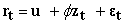(5)

where,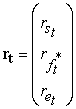,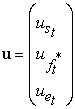,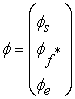,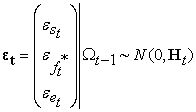,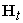is the conditional variance-covariance matrix. rt is the asset returns at time t. zt is the vector of the error correction terms.

The VEC-MGARCH model in this paper is estimated by the techniques of Diagonal BEKK, Quasi-Maximum Likelihood (QML), and the Marquardt algorithm. The BEKK GARCH parameterization, proposed by Engle and Kroner (1995), circumvents restrictions in Bollerslev et al. (1988), such as the large number of parameters to be estimated, difficulties in obtaining a stationary covariance process, and the problems in achieving a positive-definite (co)variance matrix. In order to simplify the estimation process for the variance equations, we adopt the covariance stationary specification of Ding and Engle (2001), which was also applied in e.g., De Santis and Gérard(1998).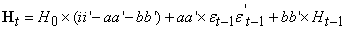where, a and b contain the diagonal elements of A and B, respectively, which are presented in Engle and Kroner (1995). H0 is the unconditional variance-covariance matrix.

In order to evaluate the performance of the different hedged portfolios, we construct the hedged portfolios based on the optimal hedge ratios for the domestic and international strategies. The IOHR hedge portfolio, estimated by the proposed approach in this study, simultaneously hedges both asset and currency risks within one operation and one set of estimated optimal hedge ratios. The IOHR hedge portfolio is compared to the traditional domestic hedge strategy, which separately hedges the asset price risk and the foreign exchange risk. For comparison purposes, we denote the domestic (or conventional) portfolio as COHR.

### 5. Data

We examined the following asset markets: 1) commodity markets: copper spot and futures markets of London Metal Exchange (LME) and New York Mercantile Exchange- Commodity Exchange (NYMEX-COMEX); 2) stock index markets: spot index and associated index futures markets of S&P the (CME); FTSE the London Stock Exchange (LSE) and London International Financial Futures Exchange (LIFFE); and the TOPIX in Tokyo Stock Exchange (TSE). These commodities and stock indices are traded in major financial markets and well studied.

For the sake of simplicity, the naming conventions of “spot-futures” markets are used to express the hedged portfolio structure with more than one currency. For example, COMEX-LME represents that the hedged portfolio consists of the copper spot asset traded in COMEX (U.S. dollar denominated) and the copper futures contract traded in LME (British pound denominated). FTSE100-TOPIX forms a hedged portfolio of the FTSE100 stock index traded in LSE as a spot asset denominated in British pounds and the TOPIX index futures contract traded in TSE as a futures asset in Japanese Yen.

We used daily, weekly and monthly data from January 1998 to June 2008 taken from Datastream, including exchange rates. USDGBP, USDJPY and GBPJPY are the exchange rates between the US dollar and the British pound, the US dollar and Japanese Yen, the British pound and Japanese Yen, respectively.

Table 1 summarizes the statistical characteristics for these assets and currencies. Augmented Dickey Fuller (ADF) tests show that asset and currency returns are stationary. The p-values of Jarque-Bera tests reject the null hypothesis of normal distributions for asset log levels, asset return series (except for the S&P 500 and TOPIX), and the currency variables (except USDGBP). The statistical results of the Ljung-Box test and the ARCH-LM test provide the evidence of the persistent autocorrelation patterns.

#### Table 1. Data Statistical Description

Table 2 shows significant correlations between currencies and assets. The correlations between currencies and futures commodity contracts are much stronger than those between currencies and stocks. This phenomenon implies that covariance between the exchange rate and the futures contract price help determine the optimal hedge ratio in Equation (4). We only report the correlations for daily data since weekly and monthly correlations are similar to daily correlation between assets and currencies.

### 6. Empirical Results

6.1. Model Estimation Results

Table 3 exhibits the results of estimated parameters from the VEC-MGARCH model. The term, μ, is statistically significant in all mean equations and horizons. The coefficients of vector error correction terms are statistically significant for both spot and futures markets, which shows the cointegration relations between international markets. The highly significant parameters (ai and bi) in the variance equations for the GARCH process indicate the significance of the second moment and make the conditional variance process clearly time-varying. Moreover, the estimates of the bi coefficients (which link second moments to their lagged value) are considerably larger than the corresponding estimates of the ai which link second moments to their past innovations (shocks). Additionally, based on the methods in Harris et al. (2007) and Andersen and Bollerslev (1998), this paper executes the robust test of conditional unbiasedness (not reported, upon request) which shows the overall satisfactory in most of the estimations.

6.2. Hedge Performance

Table 4 reports the results of hedge effectiveness measured by the variance reduction and the expected utility. The results show that all of the hedge portfolios achieve substantial variance reduction relative to the unhedged portfolios, in line with prior studies in earlier sections. Moreover, the IOHR hedge portfolios obtain a slightly larger variance reduction than the COHR hedge portfolios in most cases with a few exceptions. This empirical result supports that the simplified and simultaneous approach in this study, which hedges both asset and currency risks in one operation and with one set of optimal hedge ratios, attains at least as much effectiveness as the COHR hedge portfolios in which the currency risk is completely and separately hedged in the currency derivative markets. In the cases of the few exceptions, the IOHR hedge portfolios realize larger expected utilities than the COHR hedge portfolios. In this regard, the IOHR hedge portfolios still retain a hedge effectiveness slightly superior to the COHR hedge portfolios. On the other hand, Table 4 shows that the hedge effectiveness of the realistic situation of the POHR (COHR is an unrealistic case in which the currency risk is assumed completely hedged out. In contrast, POHR, a more realistic case, is assumed that the currency risk is partially hedged. In this paper, we assume POHR for currency risk by 90% hedge.) hedge portfolios is consistently inferior to the IOHR hedge portfolios by the comparison of both the variance reduction and the expected utility.

However, as observed in Table 5, the variance differences between the IOHR and COHR hedge portfolios are fairly minor. Thus, whether the variance differences are significant for the superiority of the IOHR hedge portfolios should be questioned. Table 5 reports the significant tests of the variance difference of each hedge portfolio relative to the IOHR hedge portfolio. The test results demonstrate that the variance differences are insignificant in most cases between the IOHR and COHR hedge portfolios, whereas significant between the IOHR and POHR hedge portfolios. This evidence challenges the validity of the superiority of the IOHR hedge portfolios to the COHR hedge portfolios, even though the IOHR hedge portfolios perform persistently better than the POHR hedge portfolios.

#### Table 5. Significant Tests of the Vairance Difference

The insignificant variance difference requests further examination of the hedge performance between these hedging strategies. Table 6 displays alternative measures of the hedge performance that reveal both the efficiency and effectiveness of a hedge portfolio. The results in Table 6 show that the IOHR hedge portfolios gain the higher hedge efficiency due to the smaller EOHR (The efficiency of optimal hedge ratios (EOHR) is defined as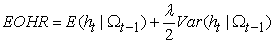) value. The better hedge efficiency implies the practical usefulness of the stable optimal hedge ratios, the lower cost, less funding, etc., for an international hedging. For instance, the smaller volatility of the estimated IOHR suggests that the IOHR hedged portfolio is capable of lowering transaction and rebalancing costs, especially compared to the traditional separate hedge strategy involving additional costs in the currency derivative markets. Likewise, in Table 5, the IOHR hedge portfolios with the smaller EER (The efficiency-effectiveness ratio (EER) is defined as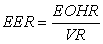where VR is the variance reduction of the hedge portfolio.) ratios exhibit better comprehensive hedge performance than the COHR hedge portfolios, despite the insignificant variance difference between the IOHR and COHR hedge portfolios. Indeed, the alternative measures of the EOHR and EER provides useful insight for the hedge performance evaluation and supplements the conventional methods.

6.3. Time-varying International Optimal Hedge Ratios

#### Table 7. Time varying optimal hedge ratio statistical description

Table 7 summarizes the statistical characteristics of the estimated time varying international optimal hedge ratio (TVIOHR). The estimated TVIOHR increases with the rise of the length of the hedge horizon, in line with the finding in Chen et al. (2004). For example, the international optimal hedge ratios of LME-COMEX hedged portfolio are 0.441, 0.779 and 0.868 for the daily, weekly and monthly horizons, respectively. The empirical results in Table VI also show that both the value and standard deviation of the TVIOHR are lower than the estimated optimal hedge ratio (OHR) of the conventional separate hedge strategy with a few exceptions in the monthly horizon. For instance, the IOHR value and standard deviation of the weekly horizon of S&P500-FTSE100 are 0.5734 and 0.135, less than those of OHR, 0.6728 and 0.159, respectively. This feature is actually consistent with the finding in the hedge efficiency based on the EOHR measurement aforementioned.

In fact, Figure 1 shows the different patterns of the dynamic optimal hedge ratios between horizons. For example, the dynamic optimal hedge ratios of the daily horizons apparently emerge the mean-reverting characteristics. The distinct movement tendency and fluctuation trend between portfolios and hedge horizons provide a hint of how investors can take advantage of the international optimal hedge ratios to construct hedge strategies. In this study, the revealed evidence supports that the results in Chen et al. (2004), which showed that both optimal hedge ratio and hedge effectiveness increase with the rise of the length of hedge horizons, are not only valid for domestic hedging, but also international hedging. Also, the insights are useful for decision-making of the hedging strategies.

Figure 1. Time-varying international optimal hedge ratios. IOHR is the international optimal hedge ratio estimated by the proposed alternative approach in this paper. OHR-CFM is the optimal hedge ratio estimated by the conventional hedge strategy in which the currency risk is hedged in currency derivative markets

### 7. Conclusions

Most prior studies have investigated hedging taking place within one domestic market. However, due to the rapid global expansion of the hedging industry and the growing demand for international hedging, more studies estimate dynamic hedge ratios in an international portfolio context. The previous researches, e.g., Bugar and Maure (2002), Larsen and Resnick (2000), and Levy and Lim (1994), among others use separate transactions in currency derivative markets to hedge currency risk. This strategy has presented issues of instable optimal solutions, determination difficulty for two sets of hedge ratios, extra input and additional costs in currency derivative markets.

However, this paper diversifies the international hedge portfolio in a different context and proposes an alternative approach to estimate the international optimal hedge ratios. The proposed approach attempts to simultaneously hedge currency risk within the same transactions in which the spot asset risk priced in the domestic currency is hedged via the futures contracts priced in the foreign currency. In other words, both asset and currency risks are hedged in one operation. As an alternative approach, this method implies that international hedging can be accomplished with one set of hedge ratios, significantly reducing the determination difficulty of estimating two sets of hedge ratios. To some extent, this strategy makes both separate transactions and extra input in currency derivative markets unnecessary, and avoids the exposure to multiple markets’ risks. Indeed, it presents a simplified approach for international hedging in practice.

The empirical result in this paper shows that the IOHR hedge portfolio attains a slightly larger variance reduction than the COHR portfolio, while its variance reduction is consistently greater than the POHR portfolio. However, the variance difference is insignificant between the IOHR and COHR hedge portfolios, whereas significant between the IOHR and POHR hedge portfolios. The insignificant variance difference generates the difficulty of comparing the superiority between IOHR and COHR hedge portfolios.

Therefore, this paper further examines their hedge performance by the efficiency and effectiveness measures. Unlike previous studies, this paper extends the hedge performance to incorporate both the hedge effectiveness and the hedge efficiency. The hedge efficiency measures the economic aspects of the hedge portfolio, such as the operation cost saving, the practicability, and the like, while the hedge effectiveness evaluates the validity of the risk-return adjustment of the hedge portfolio.

As a matter of fact, the constructed alternative measures show greater hedge efficiency and effectiveness for the IOHR hedge portfolios. The efficiency (EOHR) measurement implies that the IOHR hedge portfolios have better practicability, such as lowering transaction and rebalance costs, reducing the financing needs, etc. In addition, the efficiency-effectiveness (EER) measurement also shows better comprehensive hedge performance than the COHR hedge portfolios, despite the insignificant variance difference. Indeed, the alternative measures of the EOHR and EER provides useful insights for the hedge performance evaluation and serves as a supplement to the conventional methods. As a result, these empirical evidences support the proposed hedge approaches with certain advantages could be valid alternatives to the conventional hedge methods.

The results in this study should be very useful in practice for both companies and investors who are interested in international hedging. For instance, using the international hedge ratio, investors can simplify the international hedging process, reduce fund budgets, avoid extra capital input and separate operation in currency futures markets, as well as pursue better hedge performance. Additionally, this study shows insights for investors making decisions regarding international hedge strategies. The evidence revealed in this study supports that the results in Chen et al. (2004), which demonstrated that both optimal hedge ratio and hedge effectiveness increase with the rise of the length of hedge horizons, are not only valid for domestic hedging, but also international hedging.

### References

  Alizadeh, A., and Nomikos, N., 2004. A markov regime switching approach for hedging stock indices. The Journal of Futures Markets, , pp. 649-674In article CrossRef  Andersen, T., & Bollerslev, T., 1998, Answering the skeptics: Yes, standard volatility models do provide accurate forecasts. International Economic Review, Vol. 39, pp. 885-905.In article CrossRef  Antell, J., Vaihekoski, M., 2007. International asset pricing models and currency risk Evidence from Finland 1970-2004. Journal of Banking and Finance 31 (9), 2571-2590In article CrossRef  Bera, A. K., Garcia, P., and Roh, J. S., 1997, Estimation of Time-Varying Hedge Ratios for Corn and Soybean: Bivariate-GARCH and Random Coefficient Approaches. Agricultural Economics, Vol. 59, pp. 346-368.In article  Bohenstedt, G.W., and A.S. Goldberger, 1969. On the Exact Covariance of Products of Random Variables. American Statistical Association Journal, 64, pp. 1439-1442.In article CrossRef  Bollerslev, T., 1986, Generalized Autoregressive Conditional Heteroscedasticity. Journal of Econometric, vol. 31, No. 3, pp. 307-327.In article CrossRef  Bollerslev, T., 1990, Modeling the Coherence in Short-Run Nominal Exchange Rates a multivariate generalized ARCH model. Review of Economics and Statistics, Vol. 72, No. 3, pp. 498-505In article CrossRef  Bollerslev, T., Engle, R. F. and J. M. Wooldridge, 1988, A Capital Asset Pricing Model with Time-Varying Covariances. , Vol. 96, No. 1, pp. 116-31.In article CrossRef  Booth, G. G., P. Brockman and Y. Tse, 1998, The Relationship Between U.S. and Canadian Wheat Futures. Applied Financial Economics, Vol. , No. , pp. 73-80.In article CrossRef  Brooks, C., Henry, O. T., and Persand, G., 2002, The Effect of Asymmetries on Optimal Hedge Ratios. Journal of Business, Vol. 75 (2002), No. 2, pp. 333-352.In article  Bugar, G., Maurer, R., 2002, International Equity Portfolios and Currency Hedging: The View Point of German and Hungarian Investors. Astin Bulletin, 32, No 1, pp. 171-197.In article CrossRef  Byström, H. N. E., 2003, The Hedging Performance of Electricity Futures on the Nordic Power Exchange. Applied Economics, Vol. 35, No. 1, pp. 1-11In article CrossRef  Chakraborty, Atreya, and Barkoulas, John T., 1999. Dynamic futures hedging in currency markets. , Taylor and Francis Journals, vol. 5(4), pp. 299-314.In article  Chan-Lau, Jorge A., 2006, Foreign exchange hedging in Chile. , Vol. 12, Number 3, pp. 250-267 (18).In article  Chen, S., Lee, CF., and Shrestha, K., 2004, An empirical analysis of the relationship between the hedge ratio and hedging horizon a simultaneous estimation of the short-and long-run hedge ratios. The Journal of Futures Markets, Vol. 24, No. 4, pp. 359-386.In article CrossRef  Chou, R.Y., 1988. Volatility Persistence and Stock Valuations-Some Empirical Evidence Using GARCH. Journal of Applied Econometrics, 3, pp. 279-294.In article CrossRef  Dark, J., 2007, Basis Covergence and Long Memory in Volatility when dynamic Hedging with Futures. , Vol. 42 Issue 4, pp. 1021-1040.In article CrossRef  De Jong, A., De Room F. and Veld, C., 1997, Out-of-Sample hedging effectiveness of currency futures for alternative models and hedging strategies. Journal of Futures Markets, Vol. 17, pp. 817-837.In article CrossRef  De Santis, G., Gérard, B., 1998. How big is the premium for currency risk? Journal of Financial Economics, Vol. 49, pp. 375-412.In article CrossRef  Ding, Z., Engle, R. F., 2001, Large scale conditional covariance matrix modeling, estimation and testing. Academia Economic Papers, Vol. 29, pp. 157-184.In article  Engle, R. F., 1982, Autoregressive Conditional Heteroscedasticity with Estimates of the Variance of U.K. Inflation. Econometrica, Vol. 50, No. 4 (Jul., 1982), pp. 987-1007.In article CrossRef  Engle, R.F., Kroner, K., 1995. Multivariate simultaneous generalized ARCH. Econometric Theory, 11, pp. 122-150.In article CrossRef  Fernandez, V., 2008, Multi-period hedge ratios for a multi-asset portfolio when accounting for returns co-movement. The Journal of Futures Markets, Vol. 28, No. 2, 182-207.In article CrossRef  Floros, C., Vougas, D.V., 2007, Lead-Lag Relationship between Futures and Spot Markets in Greece 1999-2001. International Research Journal of Finance and Economics, ISSN1450-2887, No. 7In article  Gagnon, L., Lypny, G. J., and McCurdy, T. H., 1998, Hedging Foreign Currency Portfolios. Journal of Empirical Finance, , September 1998, Pp. 197-220.In article CrossRef  Gardner, Grant W. and Stone, Douglas, 1995. Estimating Currency Hedge Ratios for International Portfolios. Financial Analysts Journal, Vol. 51, No. 6, pp. 58-64.In article CrossRef  Ghosh, A., 1993, Cointegration and Error Correction Models Intertemporal Causality Between Index and Futures Prices. The Journal of Futures Markets, , Pp. 193-198In article CrossRef  Grossman, S.J., and R.J. Shiller, 1981, The Determinants of the Variability of Stock Market Prices, American Economic Review, 71, pp. 222-227.In article  Hagelin, N., Bengt Pramborg, B., 2004, Hedging Foreign Exchange Exposure Risk Reduction from Transaction and Translation Hedging. Journal of International Financial Management and Accounting, 151.In article  Harris, R.D.F., Stoja, E., and Tucker, J, 2007, A simplified approach to modeling the co-movement of asset returns. The Journal of Futures Markets, Vol. 27, No. 6, pp. 575-598.In article CrossRef  Henderson, C., 2002, Editorial Hedging emerging market currency risk. Derivatives Use, Trading & Regulation; 8, 1; ABI/INFORM Global pg. 5.In article  Hsu, Chih-Chiang, Tseng, Chih-Ping and Wang, Yaw-Huei, 2007, Dynamic Hedging with Futures A Copula-Based GARCH Model. Journal of Futures Markets, Forthcoming Available at SSRNIn article  Jacobsen, Brian J., Liu, Xiaochun, 2008, China's segmented stock market: An application of the conditional international asset pricing model. Emerging Markets Review, Vol. 9, pp 153-173.In article CrossRef  Jickling, Mark, 2006. Proposed Transaction Fee on Futures Contracts. CRS Report for Congress. Congressional Research Service, The Library of Congress. Available atIn article  Kerkvliet, J., Moffett, M.H., 1991, The Hedging of an Uncertain Future Foreign Currency Cash Flow. The Journal of Financial and Quantitative Analysis, Vol. 26, No. 4, pp. 565-578.In article CrossRef  Kooli, M., Amvella, S.P.; and Gueyie, J.P. 2005. Hedge Funds in a Portfolio Context: A Mean-Modified Value-at-Risk Framework. Derivatives Use, Trading & Regulation, 10, pp. 373-383In article  Korn, Olaf and Koziol, Philipp, 2008. The Term Structure of Currency Hedge Ratios. Available at SSRN:In article  Kroner, Kenneth F. and Sultan, Jahangir, 1993. Time-Varying Distributions and Dynamic Hedging with Foreign Currency Futures. Journal of Financial and Quantitative Analysis. Vol. 28, No 4, pp. 535-551.In article CrossRef  Larsen, G. A., Resnick, B. G., 2000, The Optimal Construction of Internationally Diversified Equity Portfolio Hedged Against Exchange Rate Uncertainty. European Financial Management, 6, pp. 479-519.In article CrossRef  Lee, H. T., Yoder, J. K., Mittelhammer, R. C., & McCluskey, J. J., 2006, A random coefficient autoregressive Markov regime switching model for dynamic futures hedging. The Journal of Futures Markets, Vol. 26, No. 2, 103-129.In article CrossRef  Lee, HT., Yoder, JK., , A Bivariate Markov Regime Switching GARCH Approach To Estimate Time Varying Minimum Variance Hedge Ratios. , Vol. 39 (2007), No. 10, Pp. 1253-1265.In article  Lee, H.T., Yoder, J., 2007b, Optimal hedging with a regime-switching time-varying correlation GARCH model. The Journal of Futures Markets, Vol. 27, No. 5, 495-516.In article CrossRef  Lee, Cheng-Few, Lee, Jang-Yi, Wang, Kehluh and Sheu, Yuan-Chung, 2008, "Estimating Future Hedge Ratio A Generalized Hyperbolic Distribution Approach", Conference on Quantitative Finance and Risk Management.In article  Leuthold, Raymond M. and Kim, Min-Kyoung, 2000, Hedging Short-term Corn Price Risks In Tokyo versus Chicago's Project A. OFOR Working Paper, No. 00-02. Available at SSRNIn article  Levy, H., Lim, K. C., 1994, Forward Exchange Bias, Hedging and the Gains from International Diversification of Investment Portfolios. Journal of International Money and Finance, 13, pp. 159-170.In article CrossRef  Lien, D.D., 2007, Optimal futures heading quadratic versus exponential utility functions. Journal of Futures Markets, Vol. 28, Number 2.In article  Lien, D. D., 1996, The Effect of the Cointegrating Relationship on Futures Hedging A Note. The Journal of Futures Markets, , Pp. 773-780.In article CrossRef  Lien, D.D. and Yang, L. 2007, Asymmetric Effects of Basis on Dynamic Futures Hedging Empirical Evidence from Commodity Markets. Journal of Banking and Finance, forthcoming.In article  Lien, D.D., Luo, X., 1993, Estimating multiperiod hedge ratios in cointegrated markets. Journal of Futures Markets, , Pp. 909-920.In article CrossRef  Lien, D.D. and Yang, Li. , "Hedging with Chinese Metal Futures". The Journal of Futures Markets, Vol. 26, No. 10, 1019-1038 (2006). Available at SSRNIn article  Lien, D.D. and Yang, Li., 2006b, spot-futures spread, time-varying correlation and hedging with currency futures. Journal of Futures Markets, Vol. 26, No. 10, pp. 1019-1038 (2006).In article CrossRef  Lien, D.D. and Yang, Li, 2008, "Asymmetric Effect of Basis on Dynamic Futures Hedging Empirical Evidence from Commodity Markets" (2007). , Vol. 32, No. 2, Pp. 187-198. Available at SSRNIn article  Lien, D.D., and Tse, YK., 1998, Hedging time-varying downside risk. Journal of Futures Markets, Vol. 18 No. 6, Pp. 705-722.In article CrossRef  Lien, D., and Tse, YK., 2000, Hedging downside risk with futures contracts. Applied Financial Economics, Vol. , No. April 2000, pp. 163-170.In article CrossRef  Lien, D., and Tse, YK., 2001, Hedging downside risk futures vs. options. International Review of Economics and Finance, Vol. 10 (2001), No. 2, Pp. 159-169.In article  Lioui, A., Poncet, P., 2002, Optimal Currency Risk Hedging. Journal of International Money And Finance, 21, pp. 241-264.In article CrossRef  Martinot, N., Lesourd, J. B., and Morard, B., 2000, On the information content of futures prices application to LME nonferrous metal futures. Working paper, University of Geneva, Switzerland.In article  McCurdy, T.H., and I. Morgan. 1988. Testing the Martingale Hypothesis in Deutsche Mark Futures with Models Specifying the Form of Heteroskedasticity. Journal of Applied Econometrics. 3, pp 187-202.In article CrossRef  Moon, Gyu-Hyen, Yu, Wei-Choun, and Hong Chung-Hyo, 2009. Dynamic hedging performance with the evaluation of multivariate GARCH models: evidence from KOSTAR index futures. Applied Economics Letters, 16, pp. 913-919.In article CrossRef  Park, T. H., and Switzer, L. N., 1995, Bivariate GARCH Estimation of the Optimal Hedge Ratios for Stock Index Futures A Note. The Journal of Futures Markets, , Pp. 61-67In article CrossRef  Power, G.J., Vedenov, D.V., 2008, The Shape of the Optimal Hedge Ratio Modeling Joint Spot-Futures Prices using an Empirical Copula-GARCH Model. NCCC-134 Conference on Applied Commodity Price Analysis, Forecasting, and Market Risk Management. St. Louis, MO. [http//www.farmdoc.uiuc.edu/nccc134].In article  Poterba, J., and L. Summers, 1986. The Persistence of Volatility and Stock Market Fluctuations. American Economic Review, 76, pp. 1142-1151.In article  Shaffer, David and A. DeMaskey. 2005. Currency Hedging Using the Mean-Gini Framework. Review of Quantitative Finance and Accounting, 25, pp. 125-137.In article CrossRef  Tong, W. H. S., 1996, An Examination of Dynamic Hedging. Journal of International Money and Finance, Vol. 15 (1996), No. 1 (February), Pp. 19-35.In article  Turvey, C.G., Nayak, G., 2003, The Semivariance-Minimizing Hedge Ratio. Journal of Agricultura1 and Resource Economics 28 (1) 100-1 15.In article  Vaihekoski, M., 2007. Global market and currency risk in Finnish stock market. Finnish Economic Papers 20 (1), 72-88.In article  Watkins, C. and McAleer, M., 2004, Econometric modeling of non-ferrous metal prices. Journal of Economic Surveys, Vol. 18, No. 5, pp. 651-701. Available at SSRNIn article  Yang, W., 2001, "M-GARCH Hedge Ratios and Hedging Effectiveness in Australian Futures Markets. Available at SSRNIn article

### Notes

1Commodity futures markets: Fernandez (2008), Power and Vedenov (2008), Hsu et al. (2007), Lien and Yang (2006a, 2008), Lien (2007), Lee et al. (2008), Lee et. al.(2006), Byström (2003), Turvey and Nayak (2003), Lien and Tse (1998, 2000, 2001), Bera et al. (1997), De Jong et. al.(1997), Martinot et. al. (2000), etc.;Foreign currency futures markets: Henderson (2002), Hagelin and Pramborg (2004), Chan-Lau (2006), Lien and Yang (2006b), etc.;Stock futures markets: Park and Switzer (1995), Tong (1996), Gagnon et al. (1998), Yang (2001), Brooks et al. (2002), Alizadeh and Nomikos (2004), Harris et al. (2007), Dark (2007), Floros and Vougas (2007), Lee and Yoder (2007a, 2007b), etc.

2See, Leuthold and Kim (2000), Booth et al. (1998), Maurer and Valiani (2004), and Kerkvliet and Moffett (1991), etc.

3See Kroner and Sultan (1993), Chakraborty and Barkoulas (1999), and Gardner and Stone (1995), etc.

4See Alizadeh and Nomikos (2004), Kooli et al. (2005), Turvey and Nayak, (2003), etc.

5See Engle (1982), Bollerslev (1986, 1990), Park and Switzer (1995), Power and Vedenov (2008), Lee and Yoder (2007), Byström (2003), Hagelin and Pramborg (2004), and Chan-Lau (2006), etc.

6See,Kroner and Sultan (1993),Lien and Yang(2007),Watkins and McAleer (2004),Martinotet al.(2000),Fung et al.(2003),Florosand Vougas(2007),Yang(2001),Lien and Yang(2006b),Dark(2007),Ghosh(1993), Lien and Luo,1993;Lien,1996, etc.